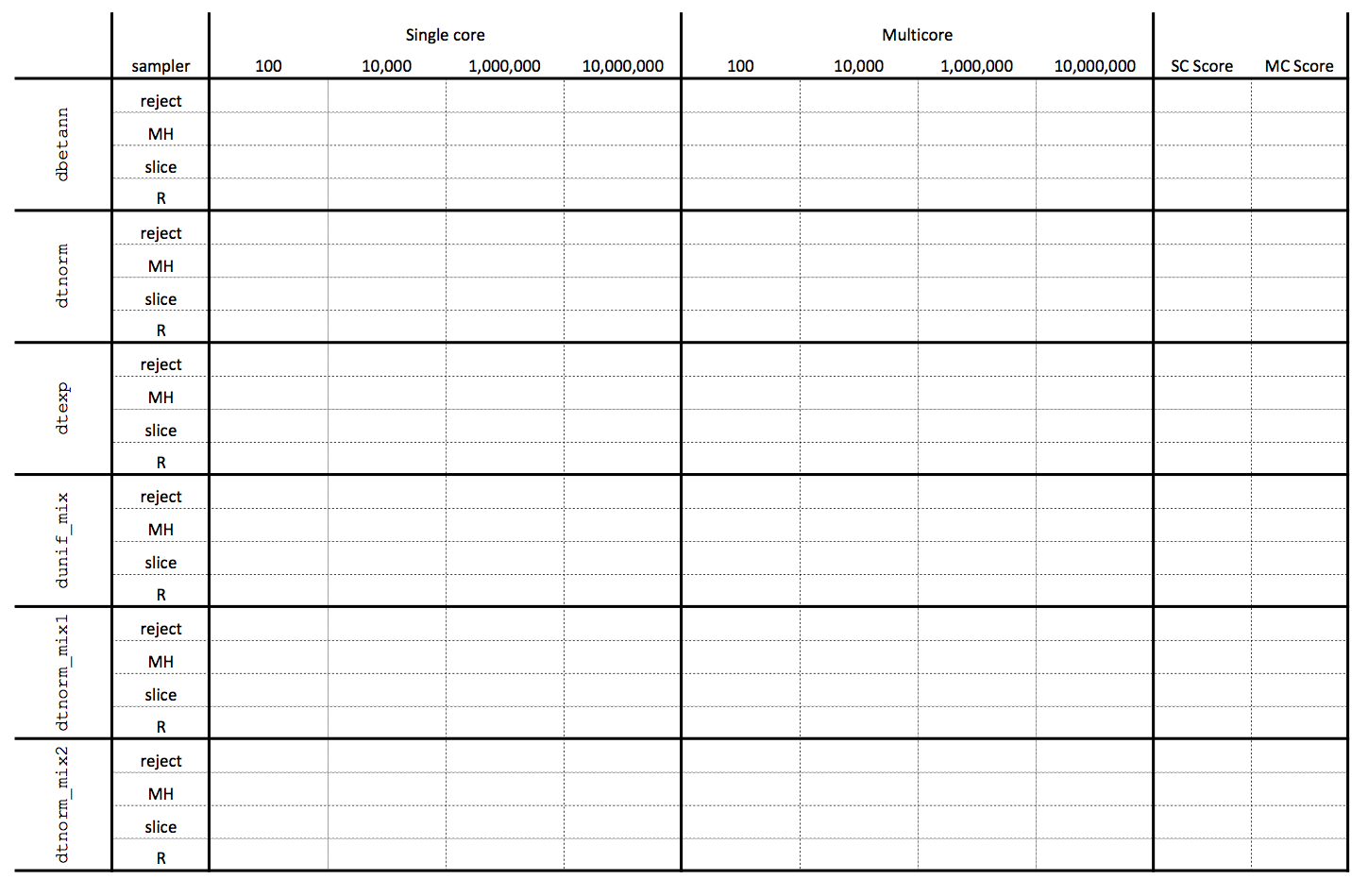## Background

For this homework you will be implementing generic functions that are able to generate samples from arbitrary distribution functions. Specifically, your team is responsible for implementing univariate sampling functions for continuous univariate probability density functions using the following approaches:

Each function must be defined as follows,

reject = function(n, dfunc, range, mc=FALSE)
{ ... }

mh = function(n, dfunc, range, mc=FALSE)
{ ... }

slice = function(n, dfunc, range, mc=FALSE)
{ ... }

R = function(n, dfunc, range, mc=FALSE)
{ ... }

where n is the number of samples, dfunc is the density function, range is a numeric vector defining the min and max range of the density function, and mc is a logical value indicating if a multicore implementation should be used. Tuning parameters for these sampling functions must be inferred automatically from the other parameters and should not be hard-coded for the test distributions. These implementations may only use the runif random number generator (you are allowed to use any RNG for the proposal distribution in the Metropolis-Hastings sampler).

Over the next two weeks we will cover several different approaches to using multiple cores for computing within R. Part of this assignment is for you to apply these techniques to your sampler implementations, how this is done will depend on the nature of the sampler (particularly depending on if the algorithm is sequential or not).

## Distributions

We will use the following 6 distributions to test the behavior and performance of the sampling algorithms defined above.

### Beta 0.9, 0.9

dbetann = function(x)
{
dbeta(x,0.9,0.9)
}### Truncated Normal

dtnorm = function(x)
{
ifelse(x < -3 | x > 3, 0, dnorm(x)/0.9973002)
}### Truncated Exponential

dtexp = function(x)
{
ifelse(x < 0 | x > 6, 0, dexp(x, rate=1/3)/0.8646647)
}### Uniform Mixture

dunif_mix = function(x)
{
ifelse(x >= -3 & x < -1, 0.6*dunif(x,-3,-1),
ifelse(x >= -1 & x <  1, 0.1*dunif(x,-1, 1),
ifelse(x >=  1 & x <  4, 0.3*dunif(x, 1, 4),
0)))
}### Truncated Normal Mixture 1

dtnorm_mix1 = function(x)
{
ifelse(x < 0 | x > 10,
0,
( 0.5*dnorm(x,mean=2,sd=2)
+0.5*dnorm(x,mean=6,sd=1))/0.9206407)
}### Truncated Normal Mixture 2

dtnorm_mix2 = function(x)
{
ifelse(x < -4 | x > 4,
0,
( 0.45*dnorm(x,mean=-4)
+0.45*dnorm(x,mean= 4)
+0.1 *dnorm(x,mean= 0,sd=0.5))/0.55)
}## Sampler Error / Scoring

The accuracy of your samplers should be tested using the following scoring function:

score = function(x, dfunc)
{
stopifnot(is.numeric(x) & length(x))

x = sort(x)
n = length(x)

ex = ecdf(x)(x)

dx = dfunc(x)
ed = cumsum(c(0, (x[-1]-x[-n])*(dx[-1]+dx[-n])/2))

return( sqrt(sum((ex-ed)^2)/n) )
}

This function calculates the square root of the average square discrepancy between the empirical CDF of the sampled values vs. the CDF of the density. The better your sampler the closer the score should be to 0, note that even a perfect sampler should not have a score of exactly 0.

## Sampler Performance

Once you have implemented your samplers the goal is to improve on them to reduce the time / sample timing across all of the test distributions. Your final write up should include a results table (like the one below) which should contain the sample/sec timing of the different samplers / sample sizes / cores.The final two columns report the results of the score function for the results of the 1,000,000 sample size condition for each condition. Only a single run of each sample size is necessary and you are welcome to use any of the profiling methods previously discussed in the class.

### Performance Contest

Like Homework 3 there will be a performance contest for this assignment. The two teams with the lowest total sum of iteration/sec timings across all conditions with be given extra credit. In order to distinguish between fast but inaccurate samplers and slow but accurate samplers the timings for each sampler will be scaled by an adjustment based on that sampler’s performance score, this scaling factor is given by the function below:

scale_time = function(score)
{
(1+score)^20
}

## Submission and Grading

This homework is due by midnight Sunday, November 23rd. You are to complete the assignment as a group and to keep everything (code, write ups, etc.) on your team’s github repository (commit early and often). All team members are expected to contribute equally to the completion of this assignment and group assessments will be given at its completion - anyone judged to not have sufficient contributed to the final product will have their grade penalized. While different teams members may have different coding backgrounds and abilities, it is the responsibility of every team member to understand how and why all code in the assignment works.